# Progressive Wave : Displacement Relation

## What Is Progressive Wave?

A wave which travels continuously in a medium in the same direction without a change in its amplitude is called a travelling wave or a progressive wave. In this section, we will derive a function that will describe the propagation of a wave in a medium and gives the shape of the progressive wave at any instant of time during its propagation.

Let us consider the example of a progressive wave on a string. Here, we will describe the relation of displacement of any element on the string as a function of time and the vibration of the elements of the string along the length at a given instant of time.

Let y(x,t) be the displacement of an element at a position x and time t about the y-axis. Considering the wave to be periodic and sinusoidal, the displacement of the element at a position x and time t from the y-axis can be given as,

y (x, t ) = a sin (kx – ωt + φ ) …………………………………..(a)

We can write the above equation as a linear combination of sine and cosine functions as,

y (x, t) =A sin (kx – ωt ) + B cos (kx – ωt ), …………(b)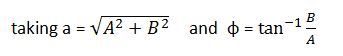The equations (a) and (b) represent the transverse wave moving along the X-axis, where y(x,t) gives the displacement of the elements of the string at a position x at any time t. Hence, the shape of the wave can be determined at any given time.

y(x, t) = a sin (kx + ωt + φ ),

The above equation represents a transverse wave moving along the negative direction of the X-axis.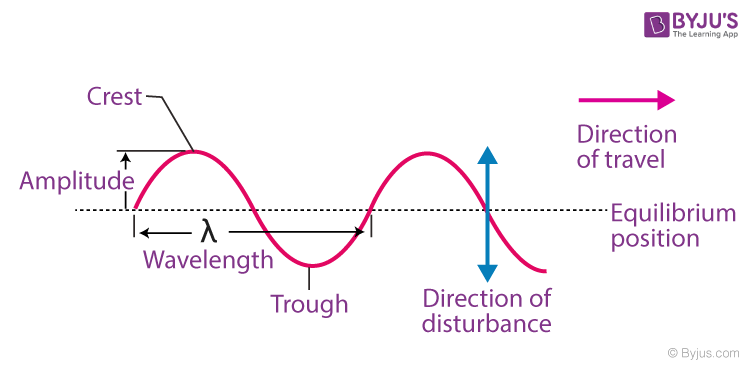The parameters that describe a harmonic wave entirely are ‘a’, ‘φ’, ‘k’, and ‘ω’, where a is the amplitude, φ is the initial phase change, k is the angular wavenumber, and ω is the angular frequency. Let us now learn in detail what these quantities represent.

Consider the sinusoidal graph shown above. Here, the plot shows a wave travelling in the positive X direction.

The point of maximum positive displacement is called a crest, and that of maximum negative displacement is called a trough.

## Amplitude

Amplitude is the magnitude of maximum displacement of a particle in a wave from the equilibrium position.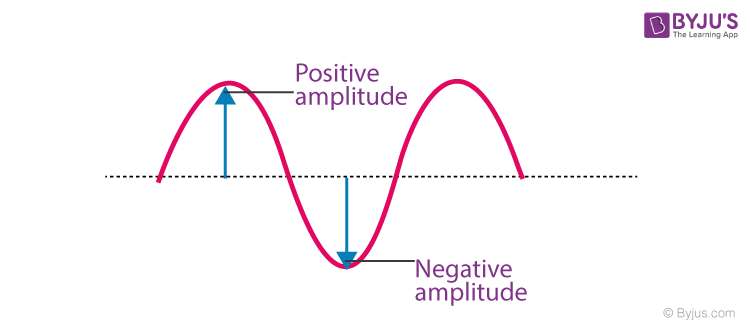The image above shows the positive and negative amplitude in the case of a sinusoidal wave. But as we consider the magnitude of the displacement, the amplitude is always a positive quantity.

## Phase

The argument (kx – ωt + φ) of the oscillatory term sin (kx – ωt + φ) is defined as the phase of the function. It describes the state of motion of the wave. Points on a wave which travel in the same direction, rising a falling together, are said to be in phase with each other. Points on a wave which travel in opposite directions to each other, such that one is rising while the other is falling, are said to be in anti-phase with each other.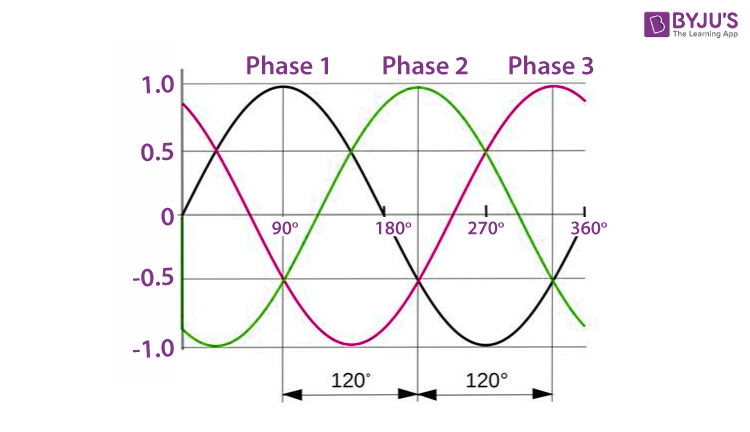## Wavelength

Wavelength (λ) is the distance between two identical points, such as a crest or a trough on a wave parallel to the wave’s propagation direction. It can also be defined as the distance over which the wave shape repeats itself. It is measured in metres (m).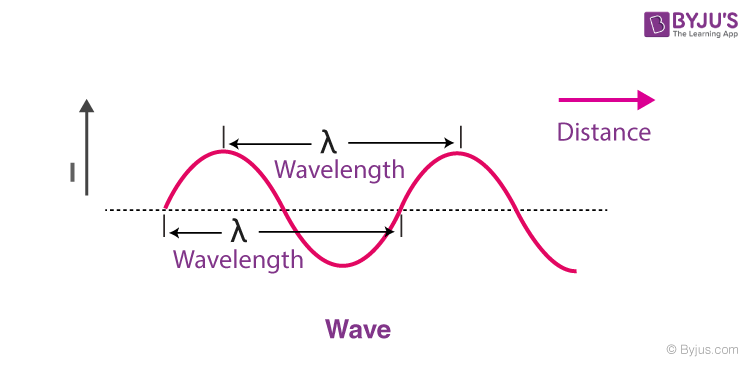### Angular wave number

The wavenumber is the spatial frequency of a wave in terms of cycles per unit distance. It can also be defined as the number of waves that exist over a specified distance, analogous to the concept of frequency.

### Angular frequency

Angular frequency is defined as the angular displacement per unit time of the rate of change of phase of a waveform.

Mathematically we can represent it as,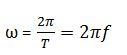where T is the time period of the sinusoidal function representing the wave and f is the frequency.

## Frequently Asked Questions – FAQs

### What are the two types of progressive waves?

There are two types of a progressive wave:
1. Longitudinal Wave
2. Transverse Waves

### Do progressive waves have a constant phase?

The amplitude of each particle is the same but the phase changes continuously

### How do particles move in a progressive wave?

A transverse wave has oscillations that are perpendicular to the direction of energy transfer whereas longitudinal oscillates parallel.

### What are the properties of progressive waves?

Properties of progressive waves are: Amplitude, wavelength, period, double periodicity, frequency and velocity.